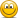# Option Pricing using binomial method

#### W0LF

##### New Member
Subscriber
Hi, I have had a quick search but not found the answer. When pricing options using the binomial method, are you supposed the discount future option pricing back to current node using relevant discount rates? I have seen an example where this is not done (the current value is just a weighted average of the future payoffs using the up/down probabilities), and this seems intuitively wrong.

Thanks.

#### David Harper CFA FRM

##### David Harper CFA FRM
Staff member
Subscriber
Hi @W0LF To my knowledge, discounting in the binomial is always with the risk-free rate; i.e., the expected future value is a weighted average of the two future nodes and this future value is discounted at the risk-free rate.
In other words, in all of the models we've built for the FRM, the node value of an option, f = exp(-Rf*ΔT)*[p*f_up + (1-p)*f_down] where Rf is the riskfree rate. That's actually the easier part, the harder part is the determination of probability, p; i.e., are they risk-neutral or real-world probabilities. In the counter-intuitive application of risk-neutral valuation (see Hull 13.2; I copied most of it below, but it's harder than it looks), the risk-free discount rate is appropriate. Hull 13.2 (emphasis mine):
13.2 RISK-NEUTRAL VALUATION: We are now in a position to introduce a very important principle in the pricing of derivatives known as risk-neutral valuation. This states that, when valuing a derivative, we can make the assumption that investors are risk-neutral. This assumption means investors do not increase the expected return they require from an investment to compensate for increased risk. A world where investors are risk-neutral is referred to as a risk-neutral world. The world we live in is, of course, not a risk-neutral world. The higher the risks investors take, the higher the expected returns they require. However, it turns out that assuming a risk-neutral world gives us the right option price for the world we live in, as well as for a risk-neutral world. Almost miraculously, it finesses the problem that we know hardly anything about the risk aversion of the buyers and sellers of options.
Risk-neutral valuation seems a surprising result when it is first encountered. Options are risky investments. Should not a person’s risk preferences affect how they are priced? The answer is that, when we are pricing an option in terms of the price of the underlying stock, risk preferences are unimportant. As investors become more riskaverse, stock prices decline, but the formulas relating option prices to stock prices remain the same.
A risk-neutral world has two features that simplify the pricing of derivatives:
1. The expected return on a stock (or any other investment) is the risk-free rate.
2. The discount rate used for the expected payoff on an option (or any other instrument) is the risk-free rate.

Returning to equation (13.2), the parameter p should be interpreted as the probability of an up movement in a risk-neutral world ... " -- Hull, John C (2014-02-19). Options, Futures, and Other Derivatives (9th Edition) (Page 278). Prentice Hall. Kindle Edition.

#### W0LF

##### New Member
Subscriber
Thanks David, this is what I thought too. In the question I was working on, the probabilities were provided as real world 50/50%. However i discounted the option intrinsic value via backward induction, however the answer did not do this. It just gave the weighted average of the future intrinsic value. Which seemed wrong to me.

#### superturtle

##### New Member
Hi @David Harper CFA FRM , I have done a search on the forum but can't find any explanation on the following statement found in part 1 notes: binomial tree chapter summary.

"This is the reason why the probability of up and down moves in the underlying does not affect the price of an option: the option is calculated in terms of the price of the underlying stock."

Seems odd to me as the binomial tree formula clearly included the probabilities. Am I missing something? Thanks in advance!

#### David Harper CFA FRM

##### David Harper CFA FRM
Staff member
Subscriber
Hi @superturtle Your comment is well-placed (ie, in the right location) because the quoted sentence (for which I did not take the time to pull up the note) is a statement about risk-neutral valuation that underling option pricing. You are correct about real-world (aka, physical) probabilities, but the binomial's risk-neutral probability is given by p\$ = [exp(r*Δt) - d] / (u - d) and this risk-neutral probability does not use the real-world probabilities per the risk-neutral framework. It follows from the idea that the option can be replicated (i.e., it binomial payoff pattern can be exactly matched) by a delta, Δ, share of the stock plus borrowing/lending at the risk-free rate; the option is replicated by a portfolio that is indifferent to the real world probabilities. It actually is oddbut very elegant. Much more depth in Hull. Thanks,